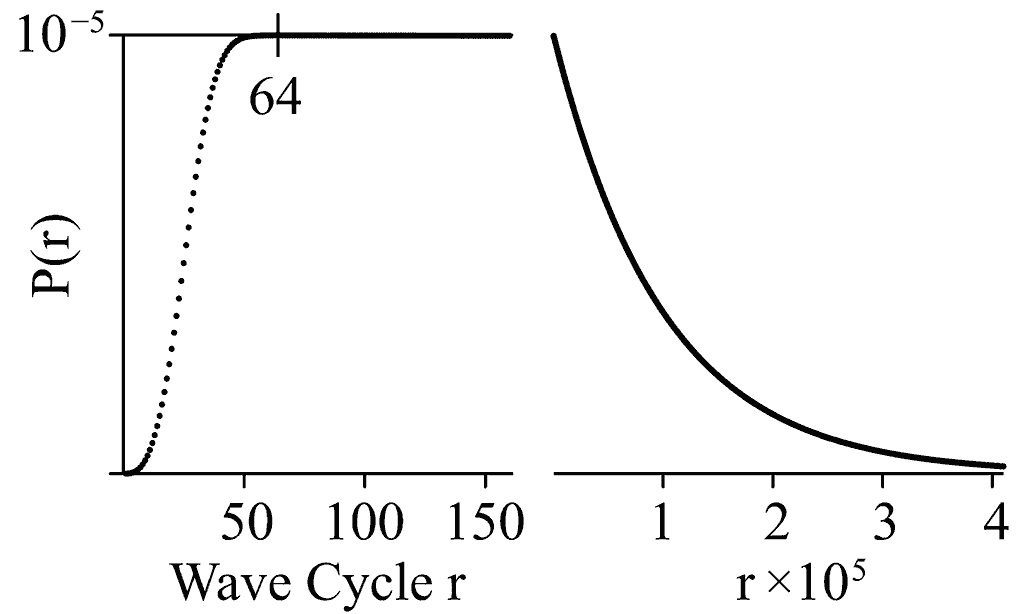Po8
Mass-Energy

# The property of intrinsic mass-energy

Mass-energy is a property of a boson, derived from the phases of its two waves. This value affects the probability that the boson will interact with others.

### Bosons as the carriers of mass-energy; creators of fermions

What we think of as creation and annihilation, actually refers to fermions, which are localized events of two bosons.

Our mechanism describes annihilation as a fermion de-constituting as radiated bosons, and creation as the formation of a fermion from bosons. Our model shows a persistent fermion to be repeatedly being created and annihilated; if it happens with the same ingredients every time, then the fermion seems to be moving.

Bosons (and therefore instances of mass-energy) are not destroyed, but they can radiate away to perhaps eventually become a part of another fermion. Only bosons having low mass-energy are likely to escape far, because massive bosons have a high probability of collapse.

The intrinsic mass-energy of a boson is a function of the phase difference of its two component waves (fig.1).Fig.1: Mass-energy ρ

Specifically, it is the cosine of the phase difference between a reference wave (having phase ${\phi }_{A}$) and its partner wave (having phase ${\phi }_{B}$). This occurs in the b basis:

(1)
$-b\rho =-b\mathrm{cos}\left({\phi }_{B}-{\phi }_{A}\right)=-b\phantom{\rule{mediummathspace}{0ex}}{e}^{-i\left({\phi }_{B}-{\phi }_{A}\right)}$

A negative mass-energy ρ results from a reference wave leading its partner by between ¼-cycle and ¾-cycle; i.e. $\rho <0$, where ρ < 0, where

(2)
π/2 < | φBφA | < 3π/2.

### The meaning of a negative value for mass-energy

This does not result in negative mass in the classical sense; in the context of gravitation, the dynamic effect is almost the same regardless of sign.

The width of the phase window for solutions is the same regardless of sign, so boson collapse (leading to a localising displacement) has approximately the same probability, but the position of the window within the phase cycle is different. This might have an effect on some phase-critical or coherent systems.

# Bosons as oscillators

The boson's waves can be interpreted as components of a simple oscillator. When these two waves are plotted on orthogonal axes, the mass-energy is equivalent to the elliptical deviation from a circle, such that a circle has a mass-energy of zero.

Mass-energy remains invariant while the boson propagates in the degenerate state, conserving its energy:

(3)
$d\rho /d\phi =0$

# Wave collapse

The mass-energy of a boson modulates the phase of any other boson that it overlaps (like a field operator), which widens the 'quantization window' for the phases of those bosons.

In QFT, We may interpret this as an excitation of the field, or an operator of the vacuum energy, so that wherever a boson is propagating, it gives mass-energy to the vacuum, as seen by other bosons.

The implication of this mechanism is that bosons having large mass tend to collapse within a short distance of their source position, and conversely, lighter bosons tend to travel further from their source position before being collapsed.

Lighter bosons (typically the flux of vacuum energy) may create quantization conditions for the more massive bosons, which provides further collapse opportunities for them. Conversely, the heavy bosons provide opportunities for the lighter bosons to collapse, when they would have otherwise propagated great distances.

# Particle size

This generalizes to a Compton radius, having a 50th percentile radius $r=\left(\mathrm{ln}2\right)/\rho$ for non-overlapping phases of a shell.

## No singularities

The above distribution has no singularity at the origin. Rather than being inversely-proportional to radius, the probability of collapse is based on the interaction of the boson's shell with a quantity of vacuum flux. In three-dimensional space, the boson's shell is the surface of a sphere, with an interaction area.

Our formulation differs form Newtonian and other classical formulations, because it does not have a singularity at radius 0, but instead falls off approaching 0. It is at this distance that Newtonian physics fails, and where ours differs from the Newtonian. This difference may be responsible for corrections like the Yukawa potential.Fig.2: Probability distribution for single iteration of collapse, with radius r: plot of ${P}_{H}\left(r\right)$ for $p={10}^{-5}$

## Degeneracy pressure

The Compton radius establishes an equilibrium distance around which particles will settle, for any given flux density of the environmental vacuum. This equilibrium does not occur in a solely Newtonian distribution. More:

# Coherence

Conserved particles are more likely to lose coherence if their bosons propagate to a large radius in the presence of a flux.

# 'Massless' photons in QED

In QED we say that photons do not have mass. Our mechanism says they may have mass, but this does not contradict QED, because our photon is transmitted as two separate bosons, which land at their target to each substitute a boson at two fermion instances at the target. This means that there is no change of mass at the target, unless the photon changes the constitution of the target fermion.

As a bonus, this substitution generates a flux from a charged particle, so we have a quantum photoelectric effect.